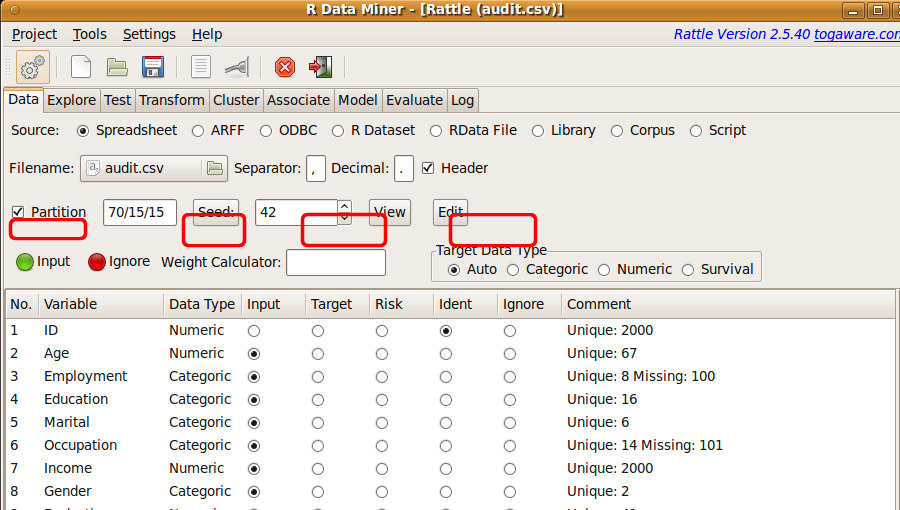DATA MINING
Desktop Survival Guide
by Graham Williams## Sampling Data

The Sample option allows us to partition our dataset into a training dataset and a testing dataset, as introduced in Section 4.1. The concepts are primarily oriented toward predictive data mining. In this case a model is built (or trained) on the training dataset. To evaluate the performance of the model we might apply the model to the testing dataset. The model has not previously been exposed to the data contained in the testing dataset, and so the performance of the model on this dataset is probably a good indication of how well the model will perform on other data as it becomes available

The concept of sampling, though, is more general than simply a mechanism for partitioning for predictive data mining purposes. Statisticians have developed an understanding of sampling as a mechanism for analysing a small dataset to make conclusions about the whole population. Thus there is much literature from the Statistics community on ensuring a good understanding of the uncertainty surrounding any conclusions we might make from analyses performed on any data. Such an understanding is important though often underplayed in the data mining context.

Rattle uses a random sampling mechanism within R, through the sample function, to perform its sample. A random sample will generally have a good chance of reflecting the distributions of the whole population. Thus, exploring the data, as we do in Chapter 6, will be made easier when very large datasets are sampled. Exploring 10,000 observations is often a more interactive and practical proposition than exploring 1,000,000 observations.

The use of sampling in this way will also be necessary in data mining when the datasets available to model are so large that the model building may take a considerable amount of time (hours or days). Sampling down to small proportions of a dataset will allow us to experiment more interactively with building a model. Once we are sure of how the data needs to be cleaned and transformed, from our initial interactions, we can start experimenting with models. After we have the basic model parameters in place we might be in a position to clean, transform, and model over a much larger portion of the data. We can leave the model building to complete over the hours that are needed.

The downside of sampling, particularly in the data mining context, is that observations that correspond to rare events might disappear from a sample. Cases of rare diseases, or of the few instances of fraud from amongst millions of electronic funds transfers, may well be lost, even though they are the items that are of most interest to us in many data mining projects. This problem is often referred to as the class imbalance problem.

One approach to the class imbalance problem is to sample our data in such a way to over-represent the rare cases that we are particularly interested in. This might use the technique of random under-sampling which will randomly choose a subset of the over-represented observations (e.g., the non-fraudulent transactions) to have, in the training dataset, something like the same number of observations as the under-represented observations. An alternative is the technique of random over-sampling, which will randomly duplicate the under-represented observations for inclusion in the training dataset. There are various tools available for sampling in R, supporting synthetic minority oversampling technique (SMOTE), cluster-based oversampling, one-sided selection, and Wilson's editing.

An alternative is to use cost sensitive learning, where the algorithm used by the model builder itself is modified. The approach introduces numeric modifications to any formula used to estimate the error in the model. Misclassifying a positive example as a negative, known as a false negative or a Type II error, (e.g., identifying a fraudulent case as not fraudulent) is sometimes more ``costly'' than a false positive or Type I error. In health, for example, we do not want to miss cases of true cancer, and might find it somewhat more acceptable to investigate cases that turn out not to be cancer, simply because missing the cancer may lead to premature death. A model-building algorithm will take into account the different costs of the outcomes and build a model that does not so readily dismiss the very much under-represented outcome.The Rattle interface provides a default random partitioning of any dataset with 70% of the data going in to a training dataset and 30% into a testing dataset (see Figure 5.11). We can override these choices, depending on our needs. A very small sampling may be required to perform some explorations of otherwise very large datasets. Smaller samples may also be required to build models using some of the more computationally expensive algorithms (like support vector machines).

Random numbers are used to select samples. Any sequence of random numbers must start with a so-called seed. If we use the same seed each time we will get the same sequence of random numbers. Thus the process is repeatable. By changing the seed we can select different random samples. This is often useful when we wish to explore the sensitivity of our models to different data samples.

Within Rattle a default seed is always used. This ensures, for example, repeatable modelling. The seed is passed to the R function set.seed to set a seed for the next generated sequence of random numbers. Thus, by setting the seed to the same number each time we can be assured of obtaining the same sample.

Conversely, we may like to set the seed to a different number in a series of model building exercises, and to then compare the performance of each model. Each model will have been built from a different random sample of the dataset. If we see significant variation between the different models, we may be concerned about the robustness of the approach we are taking. We discuss this further in Chapter.